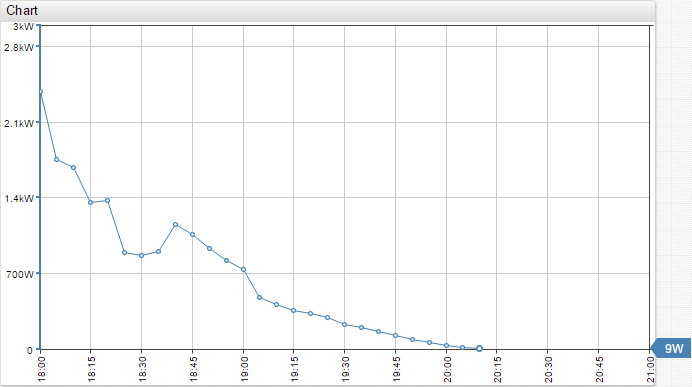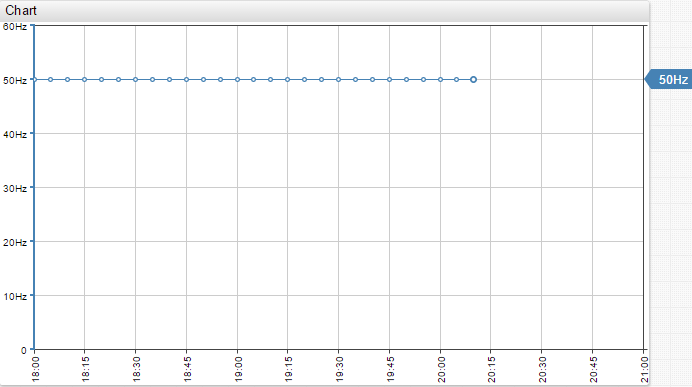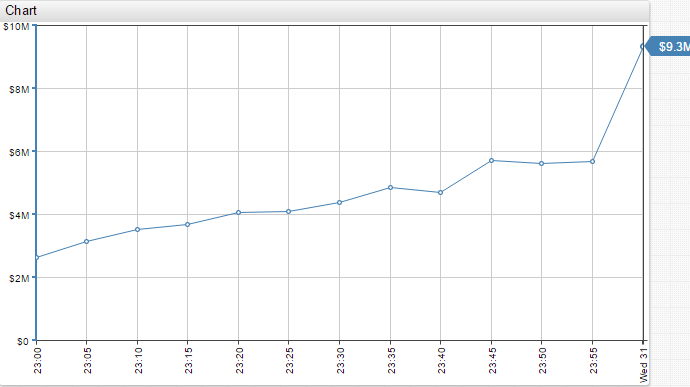# Format Settings

#### Supported format settings:

Built-in formats: bytes, watt, watt hour, hertz, joule

Built-in format examples:

```format = bytes format = kilobytes format = megawatt format = kilowatthour format = hertz format = kilojoule format = million watt format = thousands```
ValueDescriptionExample
binaryFormatting values with size units, used for bytes.
For example: 1024
`format = binary('kilo', 3) + 'B'`
decimalNumber formatting.
Numeric is an alias for decimal
in brackets an optional parameter can be specified: to which decimal is the number rounded, which digit after the comma.
For example: `format = numeric(1)` will round to the first decimal after the comma (12.28 will be rounded to 12.3).
```format = numeric(1) format = numeric```
fixedFormat numbers with the specified number of digits in the fraction portion of a number. Default – 0 fractional digits.`format = fixed(3)`
currencyCurrency formatting.`format = '\$' + currency('million')`

All functions have two optional arguments: dimension and digits.

dimension – can be set to kilo|thousand(s), mega|million(s), giga|billion(s) etc

digits – maximum amount of digits after decimal point

##### Rounding:
```format = round(0) format = round(value/512, 1) format = round(-3)```

`round([value, ] number_of_digits)`

• If `number_of_digits` is greater than 0 (zero), then the value is rounded to the specified number of decimal places.
• If `number_of_digits` is 0, then the value is rounded to the nearest integer.
• If `number_of_digits` is less than 0, then the value is rounded to the left of the decimal point.

Example: 12.34 rounded to 12.3
`format = round(1)`

Example: 12.34 rounded to 12
`format = round(0)`

Example: 12.34 rounded to 10
`format = round(-1)`

Chart Lab: View

##### Percent Format:
```format = percent format = percent(1) format = fraction format = fraction(1)```

`percent` – values will be formatted as a percentage from 100. Normally applies to values that have a scale from 0 to 100.
For example: `format = percent(1) : 10.23243232 -> 10.2%`

Chart Lab: View

`fraction` – values will be multiplied by 100 in order to convert them into a percentage. Normally applies to values that have a scale from 0 to 1.
For example: `format = fraction(2) : 0.23243232 -> 23.24%`

Chart Lab: View

`fixed([value, ] number_of_digits)` – specifies the exact number of digits in the fraction portion of a number.

Example: fixed(3)

```fixed(0, 3); // 0.000 fixed(0); // 0 fixed(1.2); // 1 fixed(1.2, 3); // 1.200 fixed(1.23, 3); // 1.230 fixed(1.234, 3); // 1.234 fixed(1.2345, 3); // 1.234 fixed(1.23456, 4); // 1.2345 fixed(0.2, 3); // 0.200 fixed(1, 3); // 1.000 fixed(1512376152, 3); // 1512376152.000 fixed(NaN, 3); // NaN fixed(null, 3); // null```
##### Time Format:
```format = iso format = new Date(value) format = (new Date(value)).toISOString()```

`iso` – print time in ISO format
`new Date(value)` – print time in current locale
`(new Date(value)).toISOString()` – print time in ISO format

Chart Lab: View

It is also possible to format time values. For example when using the `min_time_value` or `max_time_value` aggregators in table or text widgets.

```format = %d/%m %H:%M format = %H:%M:%S```

Chart Lab: View

##### Interval Format:

To format series values which represent duration in milliseconds use ‘intervalFormat’ function or ‘interval-format’ setting:

```# invoke intervalFormat function format = intervalFormat('%dd %H:%M:%S')(value*1000)   # apply the default format interval-format = true   # apply custom format interval-format = %H:%M```

The following placeholders are supported:

`%d` – day
`%H %h` – hour
`%M %m` – minute
`%S %s` – second
`%L %l` – millisecond

Chart Lab: View View View

##### Day Format:

Date format of the x-axis – year, month, week, day.
Set using a combination of the following:

`%a` – abbreviated weekday name, for example: Sun, Mon, Tue, Wed, Thu, Fri, Sat.
`%aa` – abbreviated weekday name, for example: Su, Mo, Tu, We, Th, Fr, Sa.
`%A` – full weekday name.
`%b` – abbreviated month name.
`%B` – full month name.
`%d` – zero-padded day of the month as a decimal number [01,31].
`%e` – space-padded day of the month as a decimal number [ 1,31]; equivalent to %_d.
`%j` – day of the year as a decimal number [001,366].
`%m` – month as a decimal number [01,12].
`%U` – week number of the year (Sunday as the first day of the week) as a decimal number [00,53].
`%w` – weekday as a decimal number [0(Sunday),6].
`%W` – week number of the year (Monday as the first day of the week) as a decimal number [00,53].
`%x` – date, as “%m/%d/%Y”.
`%y` – year without century as a decimal number [00,99].
`%Y` – year with century as a decimal number.

`\n` – can be added to the format string for a line break.

```day-format = %m/%d day-format = %y/%m/%d day-format = %Y/%m/%d day-format = %Y %m/%d day-format = %m %a day-format = %m/%aa day-format = %x day-format = %W\n%a```

Chart Lab: View View

##### Hour Format:

Hour format of the x-axis – hour, minute.
Set using a combination of the following:

`%H` – hour (24-hour clock) as a decimal number [00,23].
`%I` – hour (12-hour clock) as a decimal number [01,12].
`%M` – minute as a decimal number [00,59].
`%p` – either AM or PM.
`%X` – time, as “%H:%M:%S”.

`\n` – can be added to the format string for a line break.

```hour-format = %I %p hour-format = %H:%M```

Chart Lab: View

#### Examples:

`format = watt``format = hertz``format = '\$' + currency('million')`